Homework Help Question & Answers

One side concept introduced introduced in the second Bayesian lecture is the conjugate prior. Sim...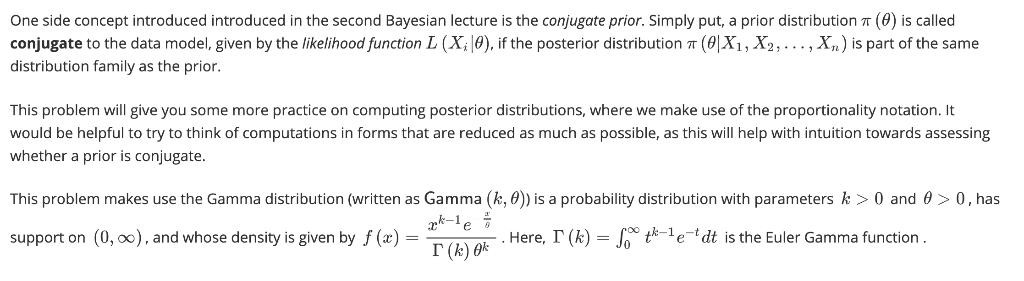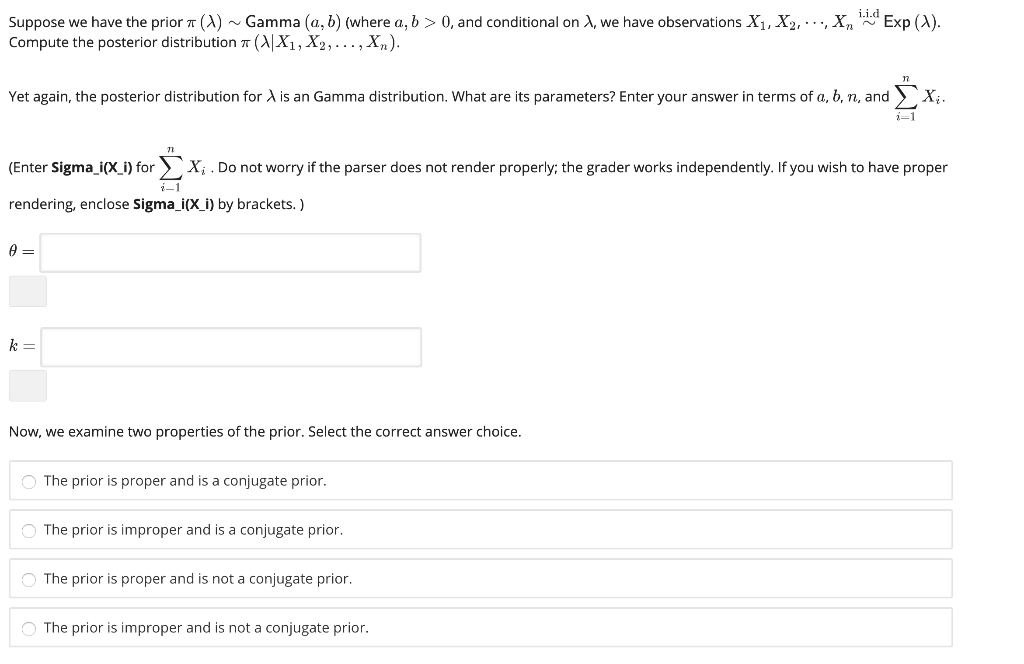One side concept introduced introduced in the second Bayesian lecture is the conjugate prior. Simply put, a prior distribution π (0) is called conjugate to the data model, given by the likelihoodfunction L (Xi θ if the posterior distribution π (ex 2, , . , X ) is part of the same distribution family as the prior. This problem will give you some more practice on computing posterior distributions, where we make use of the proportionality notation. It would be helpful to try to think of computations in forms that are reduced as much as possible, as this will help with intuition towards assessing whether a prior is conjugate. This problem makes use the Gamma distribution (written as Gamma k, θ ) is a probability distribution with parameters k > 0 and θ > 0, has T(kew Here, Г (k)-r tk-le-tdt is the Euler Gamma function Here, Г (k)-Joo tk-le-tdt is the Euler Gamma function support on (0, oo), and whose density is given by f (x) - r (h) 0k
iid Suppose we have the prior π (A) ~ Gamma (a, b) (where a, b > 0, and conditional on λ we have observations X1, X2'···Xn Compute the posterior distribution π 예 X1 , X2…·, Xn) Exp (A). 17 Yet again, the posterior distribution for λ is an Gamma distribution, what are its parameters? Enter your answer in terms of a, b, n, and 〉·X, 7t Enter Sigma-(Xi) for 〉 , Xi . Do not worry if the parser does not render properly; the grader works independently. If you wish to have proper rendering, enclose sigma i(X i) by brackets. θ= Now, we examine two properties of the prior. Select the correct answer choice. The prior is proper and is a conjugate prior. The prior is improper and is a conjugate prior. The prior is proper and is not a conjugate prior. The prior is improper and is not a conjugate prior.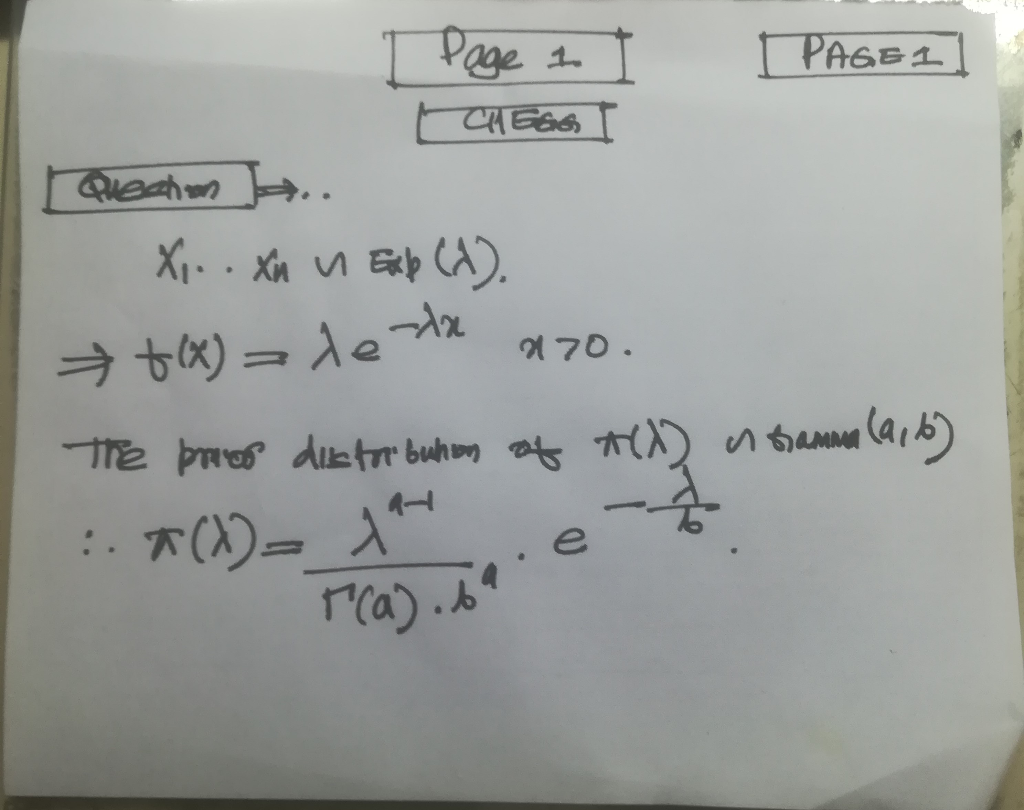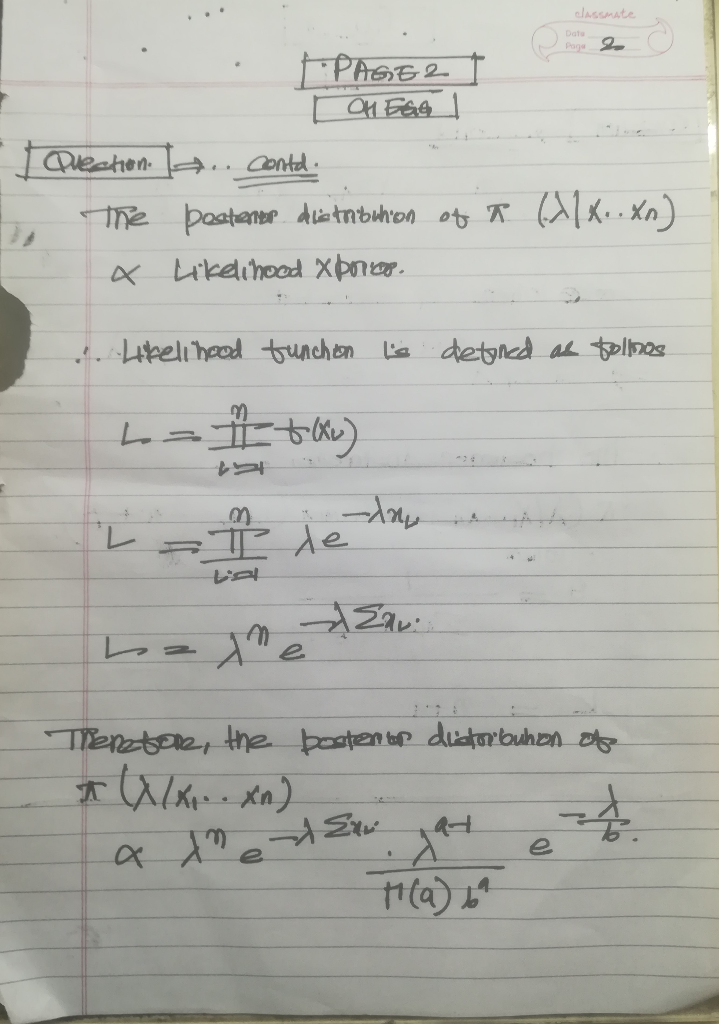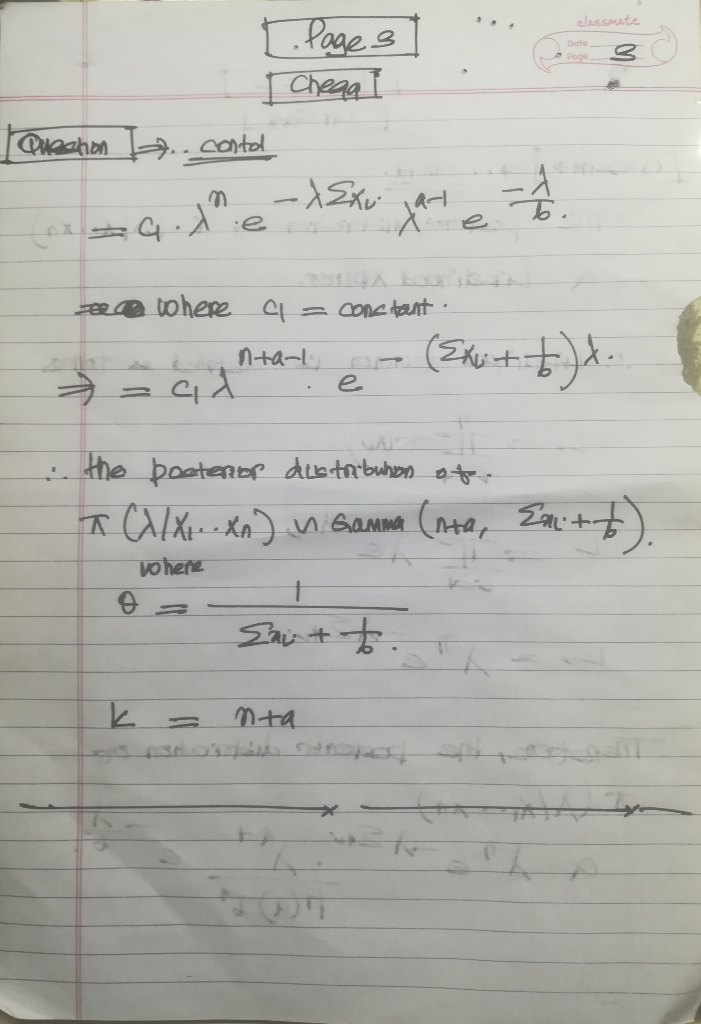Add Answer of: One side concept introduced introduced in the second Bayesian lecture is the conjugate prior. Sim...
More Homework Help Questions Additional questions in this topic.

• He second form for one-parameter exponential family distributions, introduced during lecture 09.1...

Need Online Homework Help?

Get FREE EXPERT Answers
WITHIN MINUTES
Related Questions